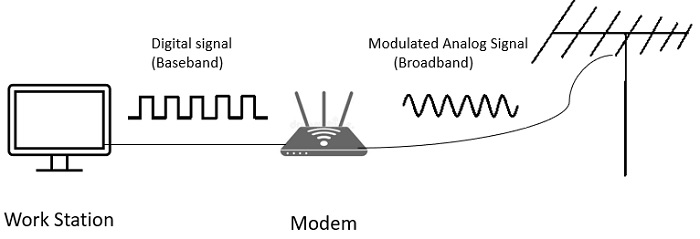# What is baseband transmission in computer networks?

Digital Modulation is the process of converting between bits and signals.

## Transmission Mechanisms

The various modulation schemes result in various transmission mechanisms which are as follows −

• Baseband transmission.
• Passband transmission.

## Multiplexing Techniques

Sharing of a transmission channel by various signals is called multiplexing. The different multiplexing techniques are as follows −

• Time division multiplexing.
• Frequency division multiplexing.
• Code division multiplexing.

Now, let us discuss one of the digital modulation schemes.

## Baseband Transmission

Baseband transmission is transmission of the encoded signal using its own baseband frequencies i.e. without any shift to higher frequency ranges. It is used for short distances.

## Steps in Baseband Transmission

Let us understand the baseband transmission step by step.

Step 1 − The most straightforward form of digital modulation is to use a positive voltage which represents ‘1’ and negative voltage represents ‘0’.

Step 2 − For an optical signal the presence of light may represent ‘1’ and absence of light represent ‘0’. This scheme is called NRZ (Non-Return-to-Zero).

Step 3 − Once sent, the NRZ signal propagates down the wire.

Step 4 − At the other end, the receiver converts it into bits by sampling the signal at regular intervals of time.

Step 5 − This signal will not look exactly like the signal that was sent.

Step 6 − It will be attenuated and distorted by the channel and noise at the receiver.

Step 7 − To decode the bits, the receiver maps the signal samples to the closest symbols.

Step 8 − For NRZ, a positive voltage will be taken to indicate that ‘1’ was sent and negative voltage will be taken to indicate that ‘0’ was sent.

Step 9 − NRZ is a good starting point for studies because it is simple.

Step 10 − In practice, it is rarely used by itself.

Step 11 − More complex schemes can convert bits to signals that better meet engineering considerations.

Step 12 − These schemes are called line codes.

Step 13 − Line codes help with −

• Bandwidth efficiency.
• Clock recovery.
• DC balance.

The baseband transmission is pictorially represented as follows −